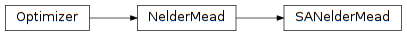# 4.8. pyopus.optimizer.sanm — Unconstrained successive approximation Nelder-Mead simplex optimizer¶Unconstrained successive approximation Nelder-Mead simplex optimizer (PyOPUS subsystem name: SANMOPT)

A provably convergent version of the Nelder-Mead simplex algorithm. The algorithm performs unconstrained optimization. Convergence is achieved by performing optimization on gradually refined approximations of the cost function and keeping the simplex internal angles away from 0. Function approximations are constructed with the help of a regular grid of points.

The algorithm was published in

 [sanm] Bűrmen Á., Tuma T.: Unconstrained derivative-free optimization by successive approximation. Journal of computational and applied mathematics, vol. 223, pp. 62-74, 2009.
class pyopus.optimizer.sanm.SANelderMead(function, debug=0, fstop=None, maxiter=None, reflect=1.0, expand=1.2, outerContract=0.5, innerContract=-0.5, shrink=0.25, reltol=1e-15, ftol=1e-15, xtol=1e-09, simplex=None, lam=2.0, Lam=4503599627370496.0, c=1e-06, tau_r=2.220446049250313e-16, tau_a=1e-100)

Unconstrained successive approximation Nelder-Mead optimizer class

Default values of the expansion (1.2) and shrink (0.25) coefficients are different from the original Nelder-Mead values. Different are also the values of relative tolerance (1e-16), and absolute function (1e-16) and side length size (1e-9) tolerance.

lam and Lam are the lower and upper bound on the simplex side length with respect to the grid. The shape (side length determinant) is bounded with respect to the product of simplex side lengths with c.

The grid density has a continuity bound due to the finite precision of floating point numbers. Therefore the grid begins to behave as continuous when its density falls below the relative(tau_r) and absolute (tau_a) bound with respect to the grid origin.

buildGrid(density=10.0)

Generates the intial grid density for the algorithm. The grid is determined relative to the bounding box of initial simplex sides. density specifies the number of points in every grid direction that covers the corresponding side of the bounding box.

If any side of the bounding box has zero length, the mean of all side lengths divided by density is used as grid density in the corresponding direction.

Returns the 1-dimensional array of length ndim holding the grid densities.

check()

Checks the optimization algorithm’s settings and raises an exception if something is wrong.

continuityBound()

Finds the components of the vector for which the corresponding grid density is below the continuity bound.

Returns a tuple (delta_prime, ndx_cont). delta_prime is the vector of grid densities where the components that are below the continuity bound are replaced with the bound. ndx_cont is a vector of grid component indices for which the grid is below the continuity bound.

grfun(x, count=True)

Returns the value of the cost function approximation at x corresponding to the current grid. If count is False the cost function evaluation happened for debugging purposes and is not counted or registered in any way.

gridRestrain(x)

Returns the point on the grid that is closest to x. The componnets of x that correspond to the grid directions for whch the density is below the continuity bound are left unchanged. The remaining components are rounded to the nearest value on the grid.

reset(x0)

Puts the optimizer in its initial state and sets the initial point to be the 1-dimensional array or list x0. The length of the array becomes the dimension of the optimization problem (ndim member).

The initial simplex is built around x0 by calling the buildSimplex() method with default values for the rel and abs arguments.

If x0 is a 2-dimensional array or list of size (ndim*+1) times *ndim it specifies the initial simplex.

A corresponding grid is created by calling the buildGrid() method.

The initial value of the natural logarithm of the simplex side vectors determinant is calculated and stored. This value gets updated at every simplex algorithm step. The only time it needs to be reevaluated is at reshape. But that is also quite simple because the reshaped simplex is orthogonal. The only place where a full determinant needs to be calculated is here.

reshape(v)

Reshapes simpex side vectors given by v into orthogonal sides with their bounding box bounded in length by lam and Lam with respect to the grid density.

Returns a tuple (vnew, logDet, l) where vnew holds the reshaped simplex sides, logDet is the natural logarithm of the reshaped simplex’s determinant, and l is the 1-dimensional array of reshaped side lengths.

logDet is in fact the natural logarithm of the side lengths product, because the reshaped sides are orthogonal.

run()

Runs the optimization algorithm.

sortedSideVectors()

Returns a tuple (vsorted, lsorted) where vsorted is an array holding the simplex side vectors sorted by their length with longest side first. The first index of the 2-dimensional array is the side vector index while the second one is the component index. lsorted is a 1-dimensional array of corresponding simplex side lengths.

#### Previous topic

4.7. pyopus.optimizer.grnm — Grid-restrained Nelder-Mead simplex optimizer

#### Next topic

4.9. pyopus.optimizer.boxcomplex — Box’s constrained simplex optimizer